2015-09-05 16:26:35 yunfeiyang62 阅读数 19214
• ###### 静态和动态控制数码管-第1季第7部分

本课程是《朱有鹏老师单片机完全学习系列课程》第1季第7个课程，全面讲解了静态数码管、无38译码器式动态数码管、有38译码器式动态数码管等各种数码管驱动方式，学完本课程将会对数码管的驱动方式彻底熟悉和掌握。

2846 人正在学习 去看看 朱有鹏

51单片机 数码管的静态显示和动态显示符号 不显示 0 1 2 3 4 5 6 7 8 9 A B C D E F . 编码 0x00 0x3F 0x06 0x5B 0x4F 0x66 0x6D 0x7D 0x07 0x7F 0x6F 0x77 0x7C 0x39 0x5E 0x79 0x71 0x80

```/*======================================================*/
/*	时间：2015年8月3日 21:19:03 						*/
/*	功能：数码管的静态显示								*/
/*	目的：51单片机学习 									*/
/*		^_^……！											*/
/*======================================================*/

#include
#include "commLib.h"

sbit WLE  = P2^7;		// 位选
sbit DLE  = P2^6;		// 段选

#define DIGITAL_ARR_NUM		18
unsigned char code digital[DIGITAL_ARR_NUM] = {				// 数码管显示数据表
/*	 0,    1,    2,    3,    4,     5,   6,    7,    8, 	*/
0x3F, 0x06, 0x5B, 0x4F, 0x66, 0x6D, 0x7D, 0x07, 0x7F,
/*	 9,    A,    B,    C,    D,    E,    F,    .,   不显示 	*/
0x6F, 0x77, 0x7C, 0x39, 0x5E, 0x79, 0x71, 0x80, 0x00
};

int main(void)
{
unsigned int i;

// 位选
WLE = 1;		// 位选端锁存器置高，送数据
P0 = 0xC0;		// 送数据
WLE = 0;		// 位选端锁存器置低，保存数据

while(1)
{
for (i = 0; i < DIGITAL_ARR_NUM; i++)
{
// 段选
DLE = 1;			// 段选端锁存器置高，送数据
P0 = digital[i];	// 送数据
DLE = 0;			// 位选端锁存器置低，保存数据

delay(500);			// 延时500ms
}
}

return 0;
}``````/*======================================================*/
/*	时间：2015年8月6日 20:45:09 						*/
/*	功能：数码管的动态显示								*/
/*	目的：51单片机学习 									*/
/*		^_^……！											*/
/*======================================================*/

#include
#include "commLib.h"

sbit WLE  = P2^7;		// 位选
sbit DLE  = P2^6;		// 段选

#define DIGITAL_ARR_NUM		18
unsigned char code digital[DIGITAL_ARR_NUM] = {				// 数码管显示数据表
/*	 0,    1,    2,    3,    4,     5,   6,    7,    8, 	*/
0x3F, 0x06, 0x5B, 0x4F, 0x66, 0x6D, 0x7D, 0x07, 0x7F,
/*	 9,    A,    B,    C,    D,    E,    F,    .,   不显示 	*/
0x6F, 0x77, 0x7C, 0x39, 0x5E, 0x79, 0x71, 0x80, 0x00
};

int main(void)
{
unsigned int i;

while (1)
{
for (i = 0; i < 6; i++)
{
P0 = 0xFF;				// 消影(必须有)
WLE = 1;				// 位选端锁存器置高，送数据
P0 = ccCrol(0xFE, i);	// 送数据
WLE = 0;				// 位选端锁存器置低，保存数据

DLE = 1;				// 段选端锁存器置高，送数据
P0 = digital[i];		// 送数据
DLE = 0;				// 位选端锁存器置低，保存数据

delay(1);
}
}

return 0;
}```2019-11-11 21:55:58 nknkkn 阅读数 67
• ###### 静态和动态控制数码管-第1季第7部分

本课程是《朱有鹏老师单片机完全学习系列课程》第1季第7个课程，全面讲解了静态数码管、无38译码器式动态数码管、有38译码器式动态数码管等各种数码管驱动方式，学完本课程将会对数码管的驱动方式彻底熟悉和掌握。

2846 人正在学习 去看看 朱有鹏

51的单片机的数码管显示

• 基于51单片机的LED数码管动态显示 LED数码管动态显示就是一位一位地轮流点亮各位数码管，对于每一位LED数码管来说，每隔一段时间点亮一次，利用人眼的“视觉暂留"效应，采用循环扫描的方式，分时轮流选通各数码管的公共端，使数码管轮流导通显示。当扫描速度达到一定程度时，人眼就分辨不出来了。尽管实际上各位数码管并非同时点亮，但只要扫描的速度足够快，给人的印象就是一组稳定的显示数据，认为各数码管是同时发光的。若数码管的位数不大于8位时，只需两个8位I／O口。

``````
```c
#include<reg52.h>
#define uchar unsigned char
#define uint unsigned int
sbit we=P2^7;
sbit du=P2^6;
sbit s2=P3^0;
sbit s3=P3^1;
sbit s4=P3^2;
uchar a,b,c,d,e,f,t,x, num,num1,num2;
uchar code yidaojiu[]={0x3f,0x06,0x5b,0x4f,0x66,0x6d,0x7d,0x07,0x7f,0x6f};
void delay(uint);
void play1(uchar,uchar,uchar,uchar,uchar,uchar);
void play2(uchar);
void main()
{
TMOD=0x01;
TH0=(65535-9216)/256;
TL0=(65535-9216)%256;
EA=1;
ET0=1;
TR0=1;
while(s2)
play1(a,b,c,d,e,f);
}
void play1(uchar a,uchar b,uchar c,uchar d,uchar e,uchar f)
{
du=1;
P0=yidaojiu[a];
du=0;
P0=0xff;
we=1;
P0=0X7f;
we=0;
delay(3);
du=1;
P0=yidaojiu[b];
du=0;
P0=0XFF;
we=1;
P0=0Xbf;
we=0;
delay(3);

du=1;
P0=yidaojiu[c];
du=0;
P0=0XFF;
we=1;
P0=0XDF;
we=0;
delay(3);
du=1;
P0=yidaojiu[d];
du=0;
P0=0xff;
we=1;
P0=0Xef;
we=0;
delay(3);
du=1;
P0=yidaojiu[e];
du=0;
P0=0xff;
we=1;
P0=0Xf7;
we=0;
delay(3);

du=1;
P0=yidaojiu[f];
du=0;
P0=0xff;
we=1;
P0=0Xfb;
we=0;
delay(3);
}
void delay(uint z)
{
uint x,y;
for(x=z;x>0;x--)
for(y=114;y>0;y--);
}
void dingshizhongduan0() interrupt 1
{
char flat;
TH0=(65535-9216)/256;
TL0=(65535-9216)%256;
if(s4==0)
flat2=0;
if(flat==0)
num++;
if(num==100)
{
num=0;
num1++;
}
if(num1==60)
{
num1=0;
num2++;
}
if(num2==100)
num2=0;
s2=1;
a=num%10;
b=num/10;
c=num1%10;
d=num1/10;
e=num2%10;
f=num2/10;
}
``````
``````
``````

2018-08-27 21:49:25 zlllg 阅读数 7479
• ###### 静态和动态控制数码管-第1季第7部分

本课程是《朱有鹏老师单片机完全学习系列课程》第1季第7个课程，全面讲解了静态数码管、无38译码器式动态数码管、有38译码器式动态数码管等各种数码管驱动方式，学完本课程将会对数码管的驱动方式彻底熟悉和掌握。

2846 人正在学习 去看看 朱有鹏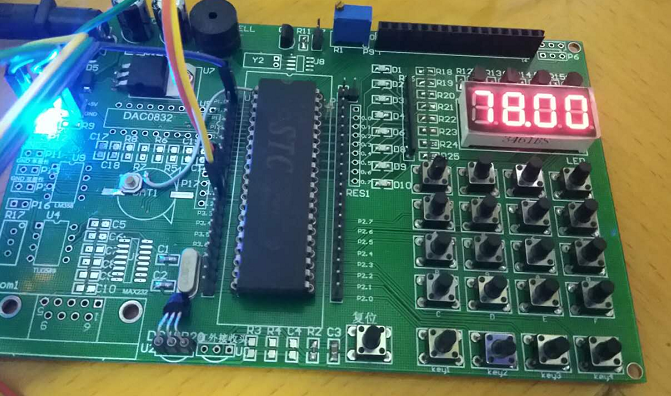C51单片机由于运行速度很慢，在高刷新频率下，单片机的资源耗费很厉害，这样单片机就不可以再进行大量的计算工作，实际上，单片机在刷新时，只需要周期性的改变GPIO口的状态就可以了，剩下的时间其实都是在空转的状态下，我们能不能将这个空转的状态拿来用呢？当然是可以的啦，这里，我们利用单片机的定时器周期地产能中断，在中断内进行数码管的刷新工作，就可以将等待中断的这个CPU时间拿来做别的事情了。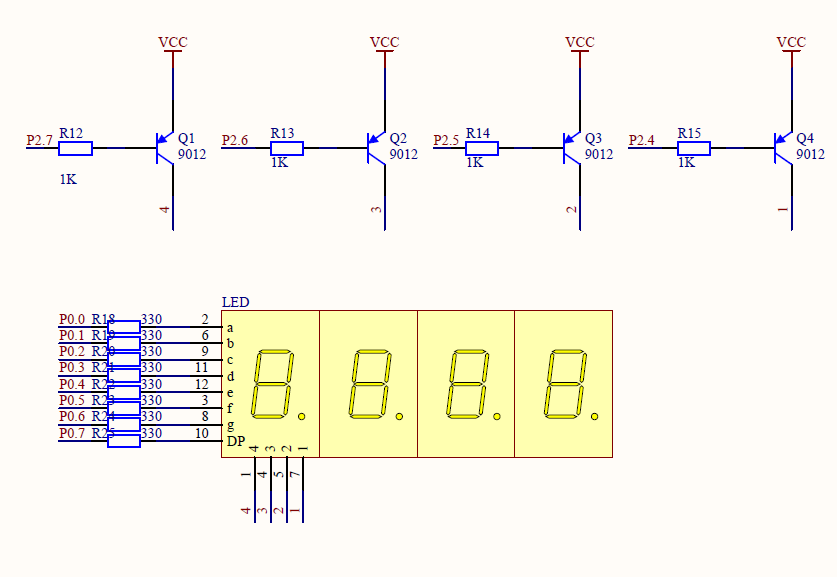``````#include "shumaguan.h"
#include "timer.h"

sbit sw_jdq=P1^0;//定义一位继电器操作
void main(void)
{
int cnt=0;//设定初值
timer_init();//初始化定时器
while(1)
{
value_now = cnt;//送初值到显示缓存
delay(50);
cnt++;
sw_jdq=~sw_jdq;//切换继电器状态
if(cnt>9999)//数值超出界线，回归最小
cnt=-999;
}
}``````

``````#include "shumaguan.h"

#define DType	1			//define the Digital LED type, 1: VCC in common, 0: GND in common
#if DType==1
/*--------------------------------------------------------
Set the digital LED display code
From left to right for
0,1,2,3,4,5,6,7,8,9,a.b,c,d,e,f,dp,-，
--------------------------------------------------------*/
uchar code DS_BUF[]={0xc0 , 0xf9 , 0xa4 , 0xb0 , 0x99 , 0x92 , 0x82 , 0xf8 , 0x80 , 0x90 , 0x88 ,
0x83 , 0xc6 , 0xa1 , 0x86 , 0x8e , 0x7f , 0xbf , 0xff };
#else
uchar code DS_BUF[]={0x3f , 0x06 , 0x5b , 0x4f , 0x66 , 0x6b , 0x7d , 0x07 , 0x7f , 0x6f , 0x77 ,
0x7c , 0x39 , 0x5e , 0x79 , 0x71 , 0x80 , 0x40 , 0x00 };
#endif
uchar xdata buf_sm;            //set display buffer

uchar frash_cnt=0;//刷新计数器
uchar wei_buf=0x10;//位输出计数器

/*--------------------------
Compute the number from Value
value: something to display, range from -999 to 9999, suport float
--------------------------*/
void calc_sm(float value)
{
uint tmp=0;
if(value>=0)//输入值为正数，不显示负号
{
if(value>9999)//输入值大于9999，显示----
{
buf_sm=17;
buf_sm=17;
buf_sm=17;
buf_sm=17;
}
else if(value>=1000)//输入值大于999，只显示整数
{
tmp=value;
buf_sm=tmp/1000;
buf_sm=tmp%1000/100;
buf_sm=tmp%100/10;
buf_sm=tmp%10;
}
else if(value>=100)//显示一位小数
{
tmp=value*10;
buf_sm=tmp/1000;
buf_sm=tmp%1000/100;
buf_sm=(tmp%100/10)+20;
buf_sm=tmp%10;
}
else if(value>=10)//显示两位小数
{
tmp=value*100;
buf_sm=tmp/1000;
buf_sm=(tmp%1000/100)+20;
buf_sm=tmp%100/10;
buf_sm=tmp%10;
}
else//显示三位小数
{
tmp=value*1000;
buf_sm=(tmp/1000)+20;
buf_sm=tmp%1000/100;
buf_sm=tmp%100/10;
buf_sm=tmp%10;
}
}
else//输入值小于0，显示负号，其余同上
{
if((value<0)&&(value>-10))
{
tmp=value*100;
tmp=abs(tmp);
buf_sm=17;
buf_sm=(tmp/100)+20;
buf_sm=tmp%100/10;
buf_sm=tmp%10;
}
else if((value<=-10)&&(value>-100))
{
tmp=value*10;
tmp=abs(tmp);
buf_sm=17;
buf_sm=(tmp/100);
buf_sm=tmp%100/10+20;
buf_sm=tmp%10;
}
else if((value<=-100)&&(value>-1000))
{
tmp=value;
tmp=abs(tmp);
buf_sm=17;
buf_sm=(tmp/100);
buf_sm=tmp%100/10;
buf_sm=tmp%10;
}

}

}
/*

*/
void display()
{
if(frash_cnt<=3)
{
wei |=0xf0;//数码管的消隐

if(buf_sm[3-frash_cnt]>=20)
{
duan = (DS_BUF[(buf_sm[3-frash_cnt])-20]&0x7f);//显示小数点
}
else
duan = DS_BUF[buf_sm[3-frash_cnt]];//不显示小数点

wei = ~wei_buf;
wei_buf <<=1;//显示位左移一位
frash_cnt++;
}
else
{
wei |=0xf0;//数码管的消隐
frash_cnt=0;
wei_buf=0x10;//显示位回到最右边
}
}
/*

*/
void delay(uint i)
{
uchar j;
for(;i>0;i--)
for(j=0;j<120;j++);

}
``````

``````#ifndef _shumaguan_h_
#define _shumaguan_h_
#include "math.h"
#include "reg52.h"
#define duan P0
#define wei P2

#define uchar unsigned char
#define uint unsigned int

extern uchar frash_cnt;
extern uchar wei_buf;

void calc_sm(float value);
void display();
void delay(uint i);

#endif
``````

``````#include "reg52.h"
#include "timer.h"
#include "shumaguan.h"

float xdata value=0 , value_now = 0;

void timer_init()
{
TMOD = 0x02;                    //set timer0 as mode1 (16-bit)
TL0 = T1MS;                     //initial timer0 low byte
TH0 = T1MS;                //initial timer0 high byte
TR0 = 1;                        //timer0 start running
ET0 = 1;                        //enable timer0 interrupt
EA = 1;                         //open global interrupt switch
}

/* Timer0 interrupt routine */
void tm0_isr() interrupt 1 using 1
{
if(value!=value_now)//检测显示值与当前值是否匹配
{
value=value_now;//刷新显示值
calc_sm(value_now);//重新计算显示值的输出缓冲区
}
display();//刷新数码管显示

}
``````

``````#ifndef _timer_h_
#define _timer_h_
#include "reg52.h"
#define uchar unsigned char
#define uint unsigned int

//-----------------------------------------------

/* define constants */
//#define FOSC 11059200L
#define FOSC 12000000L

#define T1MS 0   //1ms timer calculation method in 12T mode

//-----------------------------------------------

extern float xdata value , value_now;

void timer_init();

#endif
``````

2019-06-05 21:40:46 qq_41879767 阅读数 113
• ###### 静态和动态控制数码管-第1季第7部分

本课程是《朱有鹏老师单片机完全学习系列课程》第1季第7个课程，全面讲解了静态数码管、无38译码器式动态数码管、有38译码器式动态数码管等各种数码管驱动方式，学完本课程将会对数码管的驱动方式彻底熟悉和掌握。

2846 人正在学习 去看看 朱有鹏
`````` 计划：通过51单片机和stm32单片机实现一些组合功能想法，这是第一记，希望能坚持下去。
开发环境：STC89C52单片机普中HC6800-ES V2.0
任务目标：利用数码管实现99999999的计时与数码管逐位显示
程序分块：利用定时器中断+静态数组存储与动态刷新，存储数码管数字1-9的code char数组
定时时间以1ms按照11.052MHZ计算对应的TH0和TL0值写入，累积1s后产生中断数码管计时，
通过关闭段选，消除了重影。
接下来是主要程序代码：
``````
``````#include <reg52.h>

unsigned char code LEDCHAR[] = {0x3f,0x06,0x5b,0x4f,0x66,0x6d,0x7d,0x07,0x7f,0x6f,0x77,0x7c,0x39,0x5e,0x79,0x71};
unsigned char LEDBUFF = 0;

sbit  A  = P2^2;
sbit  B1  = P2^3;
sbit  C  = P2^4;
unsigned char i = 0;//动态扫描索引时间
unsigned int cnt = 0 ;//记录中断次数

void main()
{

unsigned long sec = 0 ;//经过时间
EA  = 1;

//由于使能位已经处于高电平使能状态故不需要激活
TMOD = 0x01;
TH0 =  0xFC;
TL0 =  0x67;//1ms计时
ET0 = 1;
TR0 = 1;
while(1)
{
if(TF0 == 1)
{
TF0 = 0;
TH0 = 0xFC;
TL0 = 0x67;
cnt++;
if(cnt >= 1000)
{
cnt = 0;
sec++;
LEDBUFF = LEDCHAR[sec%10];
LEDBUFF = LEDCHAR[sec/10%10];
LEDBUFF = LEDCHAR[sec/100%10];
LEDBUFF = LEDCHAR[sec/1000%10];
LEDBUFF = LEDCHAR[sec/10000%10];
LEDBUFF = LEDCHAR[sec/100000%10];
LEDBUFF = LEDCHAR[sec/1000000%10];
LEDBUFF = LEDCHAR[sec/10000000%10];
//可以通过该改变cnt验证位数，发现这块开发板上数码管驱动的译码器编号与其不同
}
}
}
}

void interrupt_timer0() interrupt 1
{
TH0 = 0xFC;
TL0 = 0x67;
cnt++;
P0 = 0x00;
switch(i)
{
case 0:A = 0;B1 = 0;C = 0;i++;P0 = LEDBUFF;break;
case 1:A = 0;B1 = 0;C = 1;i++;P0 = LEDBUFF;break;
case 2:A = 0;B1 = 1;C = 0;i++;P0 = LEDBUFF;break;
case 3:A = 0;B1 = 1;C = 1;i++;P0 = LEDBUFF;break;
case 4:A = 1;B1 = 0;C = 0;i++;P0 = LEDBUFF;break;
case 5:A = 1;B1 = 0;C = 1;i++;P0 = LEDBUFF;break;
case 6:A = 1;B1 = 1;C = 0;i++;P0 = LEDBUFF;break;
case 7:A = 1;B1 = 1;C = 1;i=0;P0 = LEDBUFF;break;
default:break;
}
}
``````

2019-11-28 20:16:47 qq_33486777 阅读数 21
• ###### 静态和动态控制数码管-第1季第7部分

本课程是《朱有鹏老师单片机完全学习系列课程》第1季第7个课程，全面讲解了静态数码管、无38译码器式动态数码管、有38译码器式动态数码管等各种数码管驱动方式，学完本课程将会对数码管的驱动方式彻底熟悉和掌握。

2846 人正在学习 去看看 朱有鹏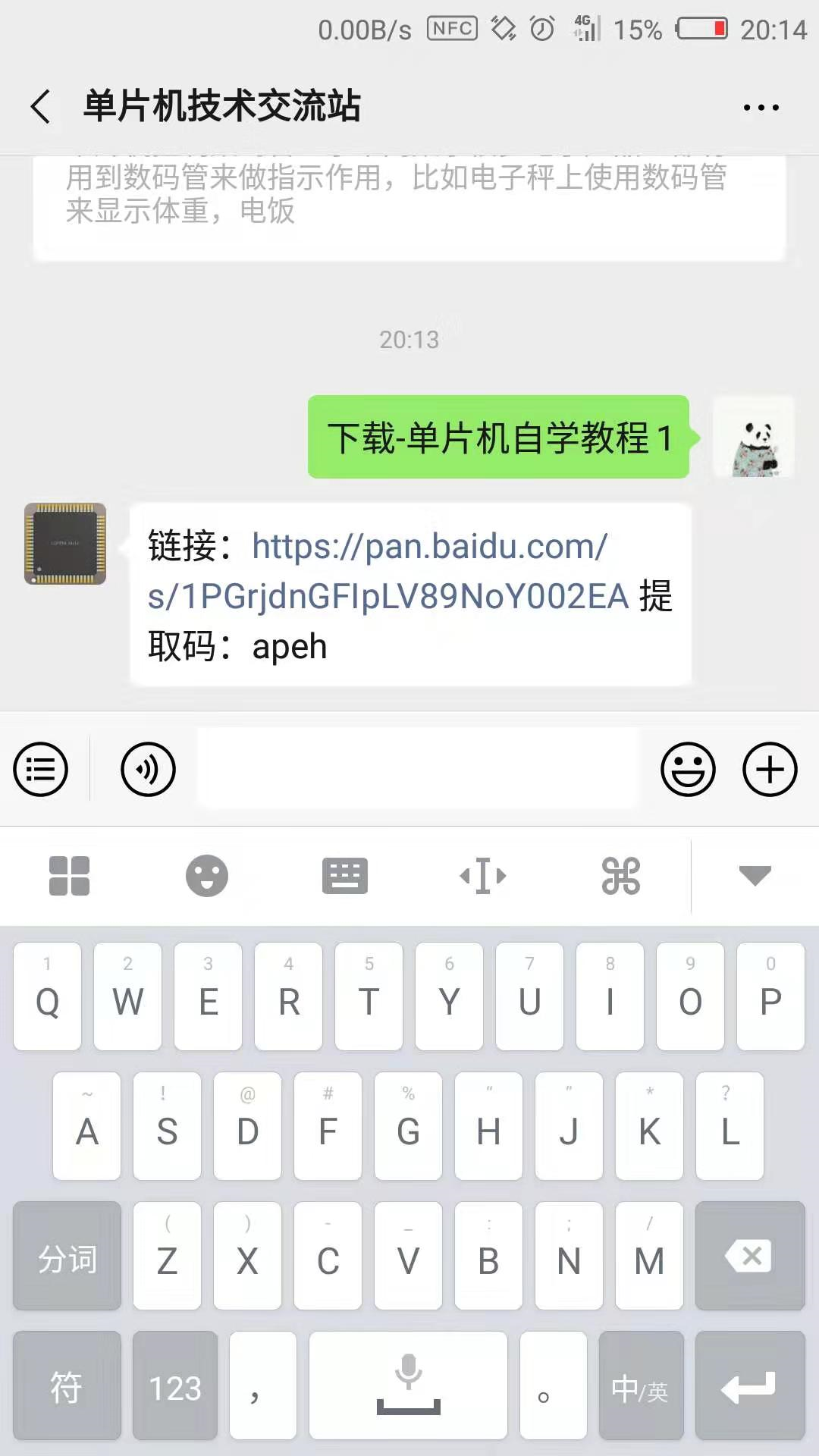想要使用单片机来控制数码管显示不同数字，那么我们先要了解数码管的内部结构以及器驱动原理。数码管可以看成是由8个发光二极管组成，我们分别给这8个数码管编号为a，b，c，d，e，f，g，SP（SP为小数点，七段数码管没有小数点），共阴极数码管就是这8个二极管的阴极连接在一起，共阳极数码管就是这8个二极管的阳极连接在一起。在控制过程中，我们把不同的二极管点亮，数码管就会给我们显示出不同的数字，例如我们把b，c两个二极管点亮，其他二极管熄灭，那么数码管显示的就是数字1；或者我们把a，b，c，d，e，f，6个数码点亮，数码管就给我们显示的是数字0.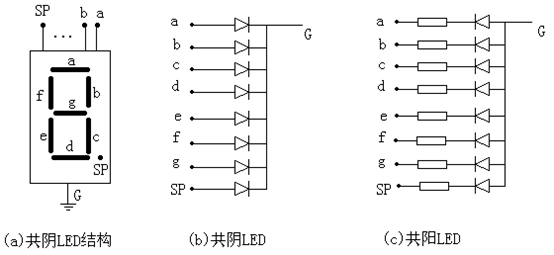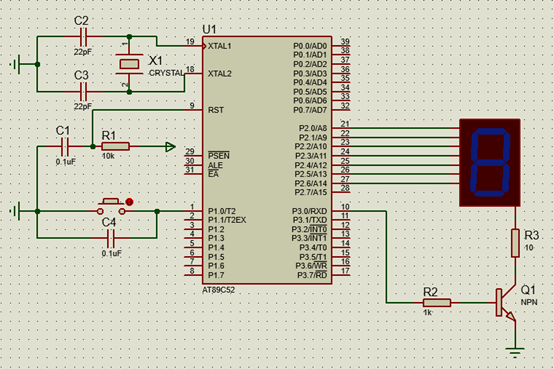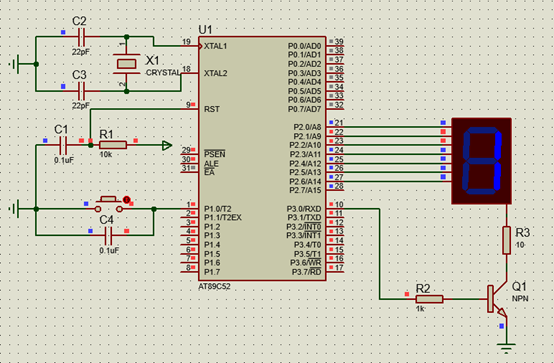SEG_ComAnodeTable[]={0x3f,0x06,0x5b,0x4f,0x66,0x6d,0x7d,0x07,0x7f,0x6f,0x77,0x7c,0x39,0x5e,0x79,0x71};

if(pTIM_Delay(0,200)) //200ms任务
{
if(SysDataVar.u8SegNumber > 15) //防止溢出
SysDataVar.u8SegNumber = 0;
LED = SEG_ComAnodeTable[SysDataVar.u8SegNumber]; //赋值
}
if(pTIM_Delay(1,5)) //5ms任务
{
Key_Sanf(); //按键检测
}